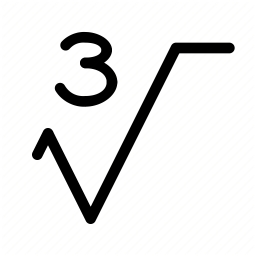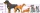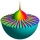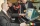# One third power

Which equation justifies why ten to the one-third power equals the cube root of ten?

x =  0

### Step-by-step explanation:Did you find an error or inaccuracy? Feel free to write us. Thank you!Math student
The step of doing ((-3)7)4## Related math problems and questions:

• Standard deviationFind standard deviation for dataset (grouped data): Age (years) No. Of Persons 0-10 15 10-20 15 20-30 23 30-40 22 40-50 25 50-60 10 60-70 5 70-80 10
• Statistical XY fileYear; money spent on advertising; profit (three values each row) 2008 2 12 2009 5 20 2010 7 25 2011 11 26 2012 15 40 1. draw a scatter diagram depicting the data. 2. calculate the Pearson's correlation coefficient. 3. determine the linear regression equat
• Linear combination of complexIf z1=5+3i and z2=4-2i, write the following in the form a+bi a) 4z1+6z2 b) z1*z2
• Std-deviationCalculate standard deviation for file: 63,65,68,69,69,72,75,76,77,79,79,80,82,83,84,88,90
• Evaluate 5Evaluate expression x2−7x+12x−4 when x=−1
• Sum of fractionsWhat is the sum of 2/3+3/5?
• ComplaintsThe table is given: days complaints 0-4 2 5-9 4 10-14 8 15-19 6 20-24 4 25-29 3 30-34 3 1.1 What percentage of complaints were resolved within 2weeks? 1.2 calculate the mean number of days to resolve these complaints. 1.3 calculate the modal number of day
• Eq2 2Solve following equation with quadratic members and rational function: (x2+1)/(x-4) + (x2-1)/(x+3) = 23
• Turtles 2A box turtle hibernates in the sand at 11 5/8. A spotted turtle hibernates at 11 16/25 feet. Which turtle is deeper? Write answer as number 1 or 2.
• Sum of mixedThe sum of two mixed numbers is 15½. One of the number is 5 ¾, what is the other?
• BoatsThree-quarters of boats are white, 1/7 are blue and 9 are red. How many boats do we have?
• Simplify 3Simplify mixed numerals expression: 8 1/4- 3 2/5 - (2 1/3 - 1/4) Show your solution.
• Mixed numbersRewrite mixed numbers, so the fractions have the same denominator: 5 1/5 - 2 2/3
• Fraction + eqSolve following simple equation with fractions: -5/6(8+5b) = 75 + 5/3b
• Subtracting complex in polarGiven w =√2(cosine (p/4) + i sine (pi/4) ) and z = 2 (cosine (pi/2) + i sine (pi/2) ), what is w - z expressed in polar form?
• Box and whisker plotConstruct a box and whisker plot for the given data. 56, 32, 54, 32, 23, 67, 23, 45, 12, 32, 34, 24, 36, 47, 19, 43
• Watching TVOne evening 2/3 of students watch TV. Of those students, 3/8 watched a reality show. Of the students that watched the show, 1/4 of them recorded it. What fraction of the students watched and recorded reality tv.